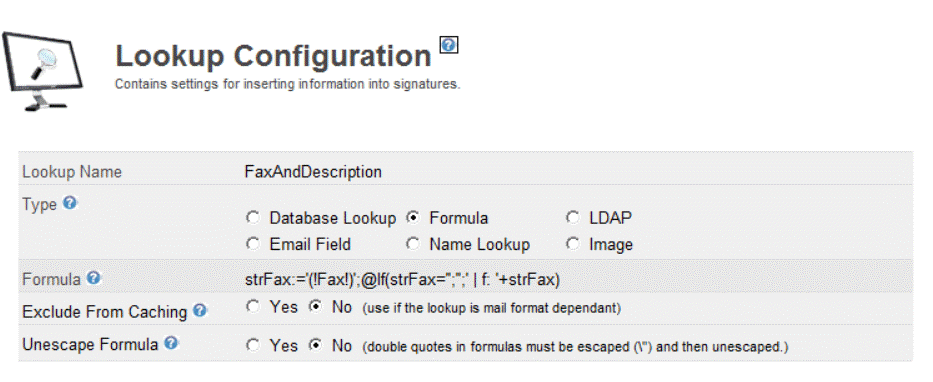Views:

Formula lookups allow you to extend the simple database lookup functions, and use the Notes `@Formula` language to evaluate a formula and return a string.### Formula

A Lotus Notes Formula that will be executed.

NOTE: The formula must not contain @DB Commands.

NOTE: All text must use single quotes, if the returned text will contain a single quote, e.g. Surnames, they you must select the "Unescape Formula" and "escape" the double quotes.

NOTE: The formula must return TEXT, e,g use the @Text() function.

The formula is parsed in the same way lookups are in the body of the signature.
In the above example the `(!Fax!)` lookup will be calculated and the results will be inserted between the single quotes to generate a string. This text is then used in the formula to determine if the fax number and description should be inserted into the signature.

Related Products: CMS DOM v4